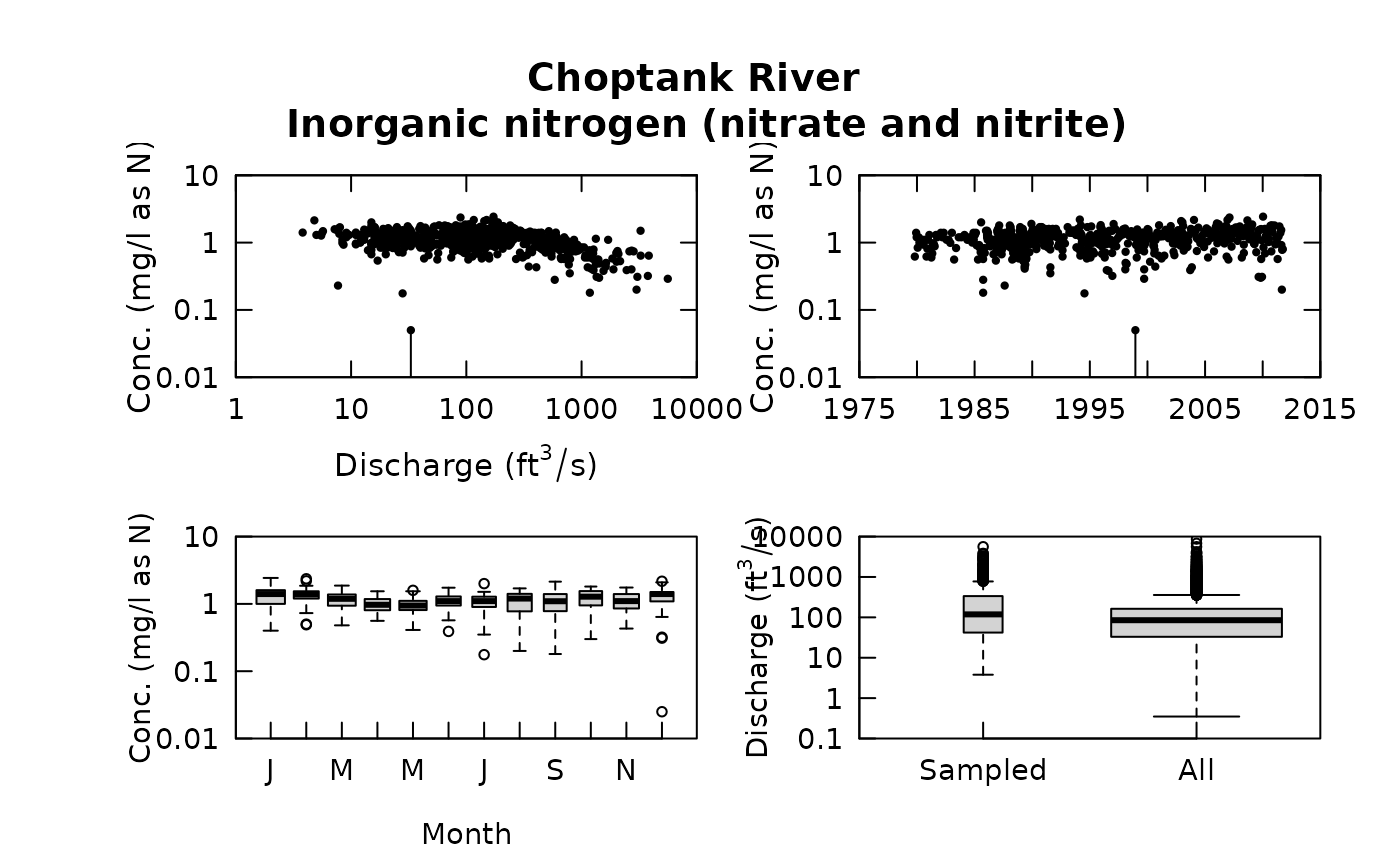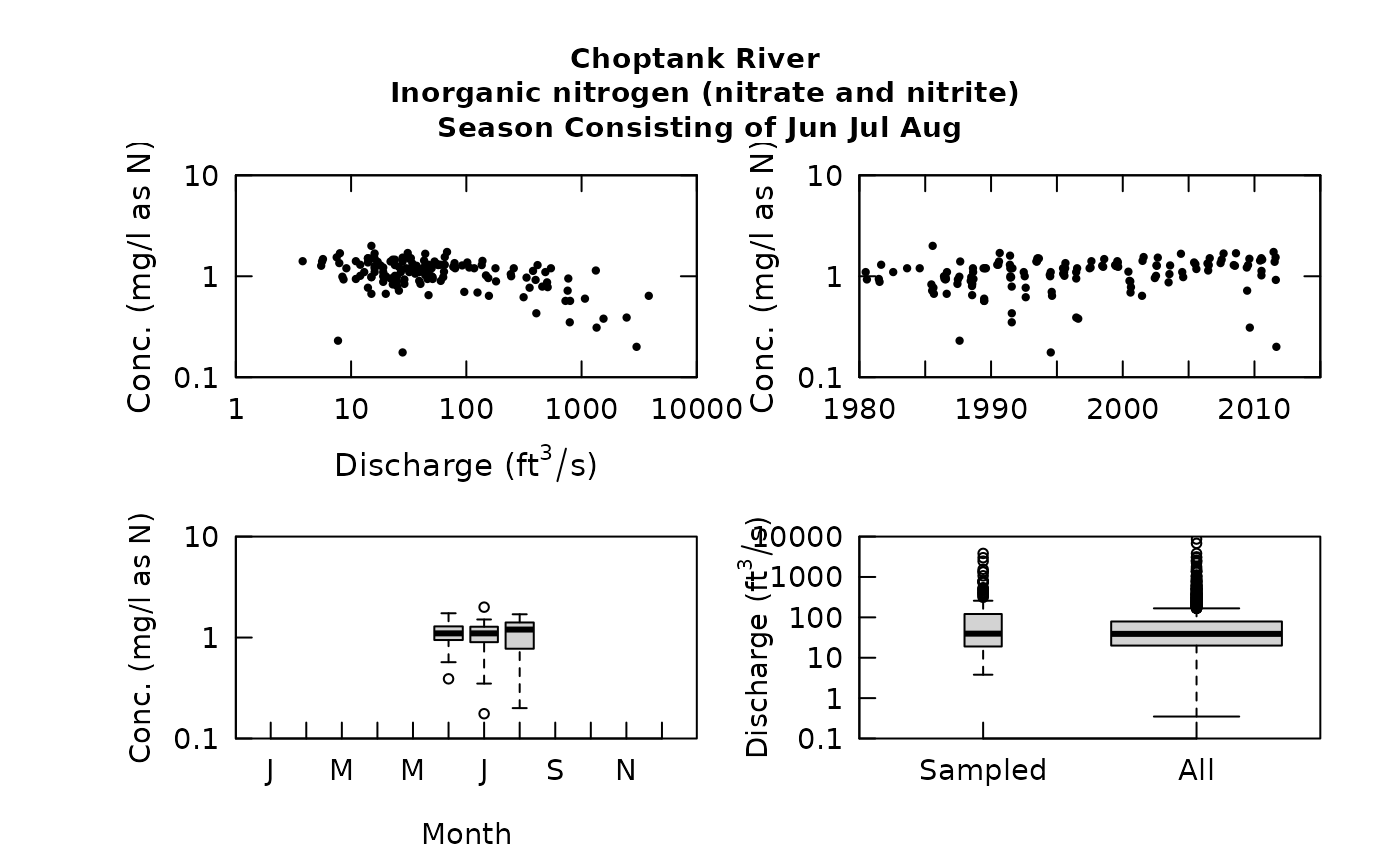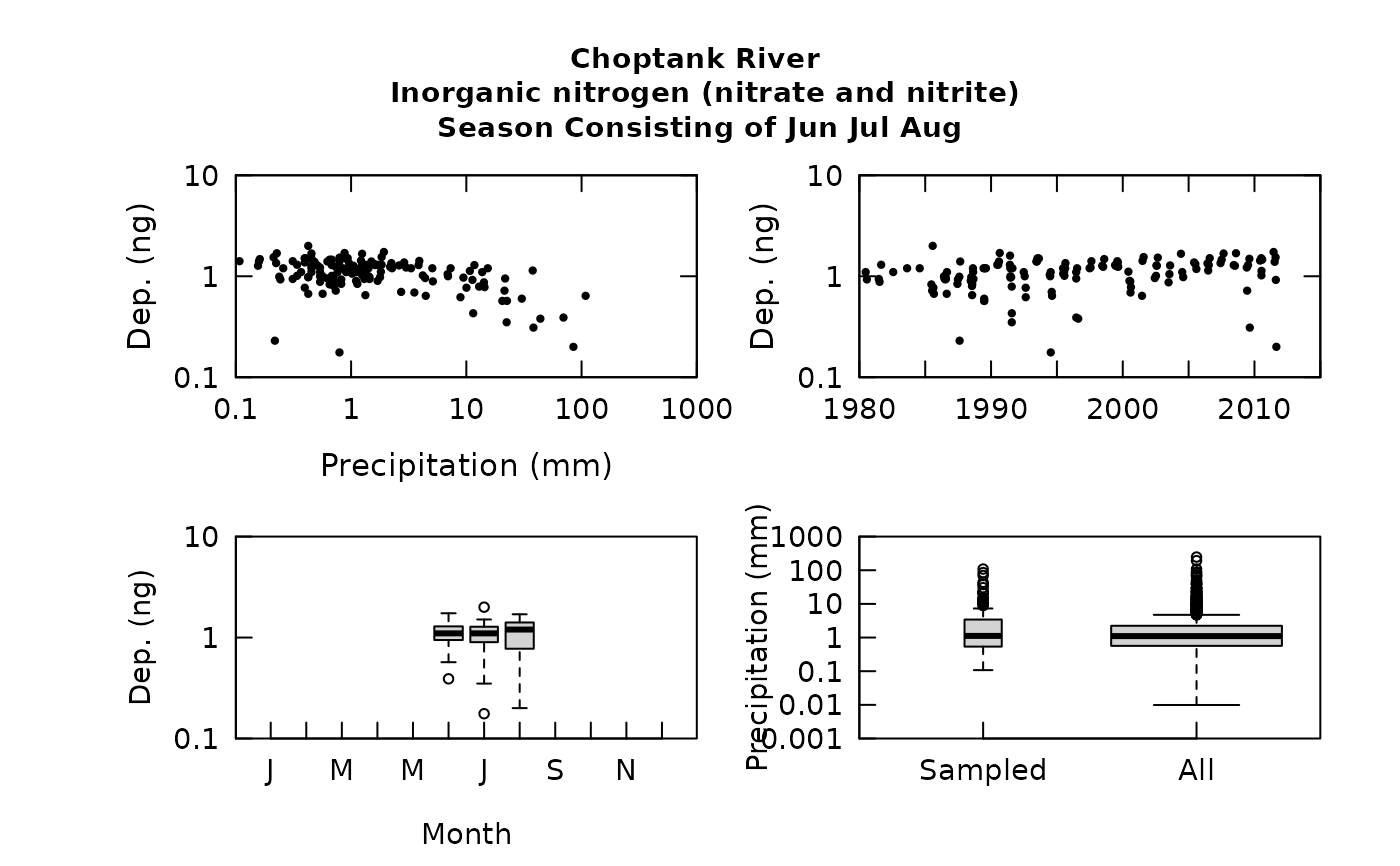This function produces the 4 plots based only on the data stored in the eList. The four plots are 1) log concentration versus log discharge, 2) log concentration versus time 3) a boxplot of log concentration by month, and 4) a side-by-side boxplot of the sampled discharges and all daily discharges. To save space, the graphic is labeled only at the top of the 4 graph display.

Although there are a lot of optional arguments to this function, most are set to a logical default.

## Usage

multiPlotDataOverview(eList, qUnit = 2, cex.main = 1.2,
randomCensored = FALSE, logScaleConc = TRUE, logScaleQ = TRUE,
concLab = 1)

## Arguments

eList

named list with at least Daily, Sample, and INFO dataframes

qUnit

object of qUnit class printqUnitCheatSheet, or numeric represented the short code, or character representing the descriptive name.

cex.main

magnification to be used for main titles relative to the current setting of cex

randomCensored

logical. Show censored values as randomized. Default is FALSE. If TRUE, makeAugmentedSample must be run first.

logScaleConc

logical if TRUE y in concentration graphs plotted in log axis. Default is TRUE.

logScaleQ

logical if TRUE y in streamflow graphs plotted in log axis. Default is TRUE.

concLab

object of concUnit class, or numeric represented the short code, or character representing the descriptive name. By default, this argument sets concentration labels to use either Concentration or Conc (for tiny plots). Units are taken from the eList$INFO$param.units. To use any other words than "Concentration" see vignette(topic = "units", package = "EGRET").

plotConcQ, boxConcMonth, plotConcTime, boxQTwice

## Examples

eList <- Choptank_eList
# Water year:
multiPlotDataOverview(eList, qUnit=1)# Graphs consisting of Jun-Aug
eList <- setPA(eList, paStart=6,paLong=3)
multiPlotDataOverview(eList, qUnit=1)# Custom axes:
eList$INFO$param.units <- "ng"
qConst_precip <- new("qUnit",
qShortName = "   mm  ",
qUnitFactor = 1,
qUnitName = "Millimeter",
qUnitExpress = expression(paste("Precipitation in ",mm)),
qUnitTiny = expression(paste("Precipitation ", "(", mm, ")")),
shortCode = 1,
unitUSGS = "Precipitation, in mm",
prefix = "Precipitation")

deposition <- new("concUnit",
longPrefix = "Deposition",
shortPrefix = "Dep")

multiPlotDataOverview(eList,
qUnit = qConst_precip,
concLab = deposition)